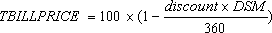# TBILLPRICE function: Description, Usage, Syntax, Examples and Explanation

## What is TBILLPRICE function in Excel?

REPT function is one of Financial functions in Microsoft Excel that returns the price per \$100 face value for a Treasury bill.

## Syntax of TBILLPRICE function

TBILLPRICE(settlement, maturity, discount)

The TBILLPRICE function syntax has the following arguments:

• Settlement: The Treasury bill’s settlement date. The security settlement date is the date after the issue date when the Treasury bill is traded to the buyer.
• Maturity: The Treasury bill’s maturity date. The maturity date is the date when the Treasury bill expires.
• Discount: The Treasury bill’s discount rate.

Note: Dates should be entered by using the DATE function, or as results of other formulas or functions. For example, use DATE(2008,5,23) for the 23rd day of May, 2008. Problems can occur if dates are entered as text.

## TBILLPRICE formula explanation

• Microsoft Excel stores dates as sequential serial numbers so they can be used in calculations. By default, January 1, 1900 is serial number 1, and January 1, 2008 is serial number 39448 because it is 39,448 days after January 1, 1900.
• Settlement and maturity are truncated to integers.
• If settlement or maturity is not a valid date, TBILLPRICE returns the #VALUE! error value.
• If discount ≤ 0, TBILLPRICE returns the #NUM! error value.
• If settlement > maturity, or if maturity is more than one year after settlement, TBILLPRICE returns the #NUM! error value.
• TBILLPRICE is calculated as follows:where:
• DSM = number of days from settlement to maturity, excluding any maturity date that is more than one calendar year after the settlement date.

## Example of TBILLPRICE function

Steps to follow:

1. Open a new Excel worksheet.

2. Copy data in the following table below and paste it in cell A1

Note: For formulas to show results, select them, press F2 key on your keyboard and then press Enter.

You can adjust the column widths to see all the data, if need be.

 Data Description 3/31/2008 Settlement date 6/1/2008 Maturity date 9.0% Percent discount rate Formula Description Result =TBILLPRICE(A2,A3,A4) The price for the Treasury bill, using the terms in A2, A3, and A4. \$ 98.45